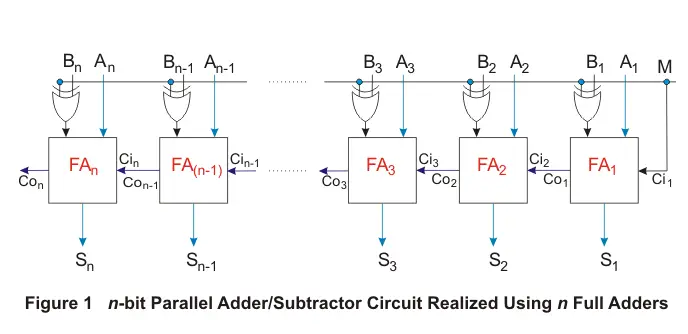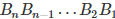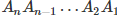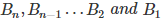We have seen parallel adder circuit built using a cascaded combination of full adders in the article Parallel Adder. Likewise in the article on Parallel Subtractor we have seen two different ways in which an n bit parallel subtractor can be designed. However always from the point of optimization, we prefer using a single circuit to accomplish multiple kinds of operations. This means in our case, we have to think whether it is possible to perform both n bit addition as well as n bit subtraction using a single digital circuit i.e. a parallel adder or subtractor circuit.
The answer is yes. This is because, the subtraction process of binary numbers is nothing but their 2’s complement addition.

Hence the design of parallel adder when manipulated in a way so as to provide the 2’s complement of the number whenever necessary can be used as a parallel adder-cum-subtractor. One such design accomplished using the XOR gates is as shown by Figure 1.Here M-line acts as a control line i.e. depending on the value provided at M, the circuit behaves either as an adder or as a subtractor. The reason for this can be explained below. Suppose, if M-line is driven low, then one of the input to each and every XOR gate would be logic 0. This means that the XOR outputs in this case will be unaltered binary bits of the number. In addition, if M = 0, the carry in pin (Ci1) of the first full adder (FA1) would also be 0. Due to these conditions, the circuit shown will be behave as a n-bit adder adding the numberwith.
Similarly let us now analyze the case where M = 1, meaning which the M control line is pulled high. In this case, one of the inputs to each XOR gate would be logic 1. This means that we get the complement of the bitsas the outputs of each XOR gate.

This indicates that the binary number is complemented before being fed as an input to each of the full adder in the circuit. In addition, for the same case, even the Ci1 of the first full adder FA1 would be logically high. As a result, the cascaded arrangement of full adders shown in Figure 1 effectively performsn-bit binary subtraction wherein the binary numberis subtracted from.

## Drawback of Parallel Adder or Subtractor

As the architecture of parallel adder or subtractor is very similar to that of a parallel adder (and also to that of a parallel subtractor), even this design is prone to the effect of ripple propagation delay. Nevertheless, these kind of circuits find their application in the field of computers as a part of arithmetic and logic (ALU) unit, aiding numerous computations.

Want To Learn Faster? 🎓
Get electrical articles delivered to your inbox every week.
No credit card required—it’s 100% free.